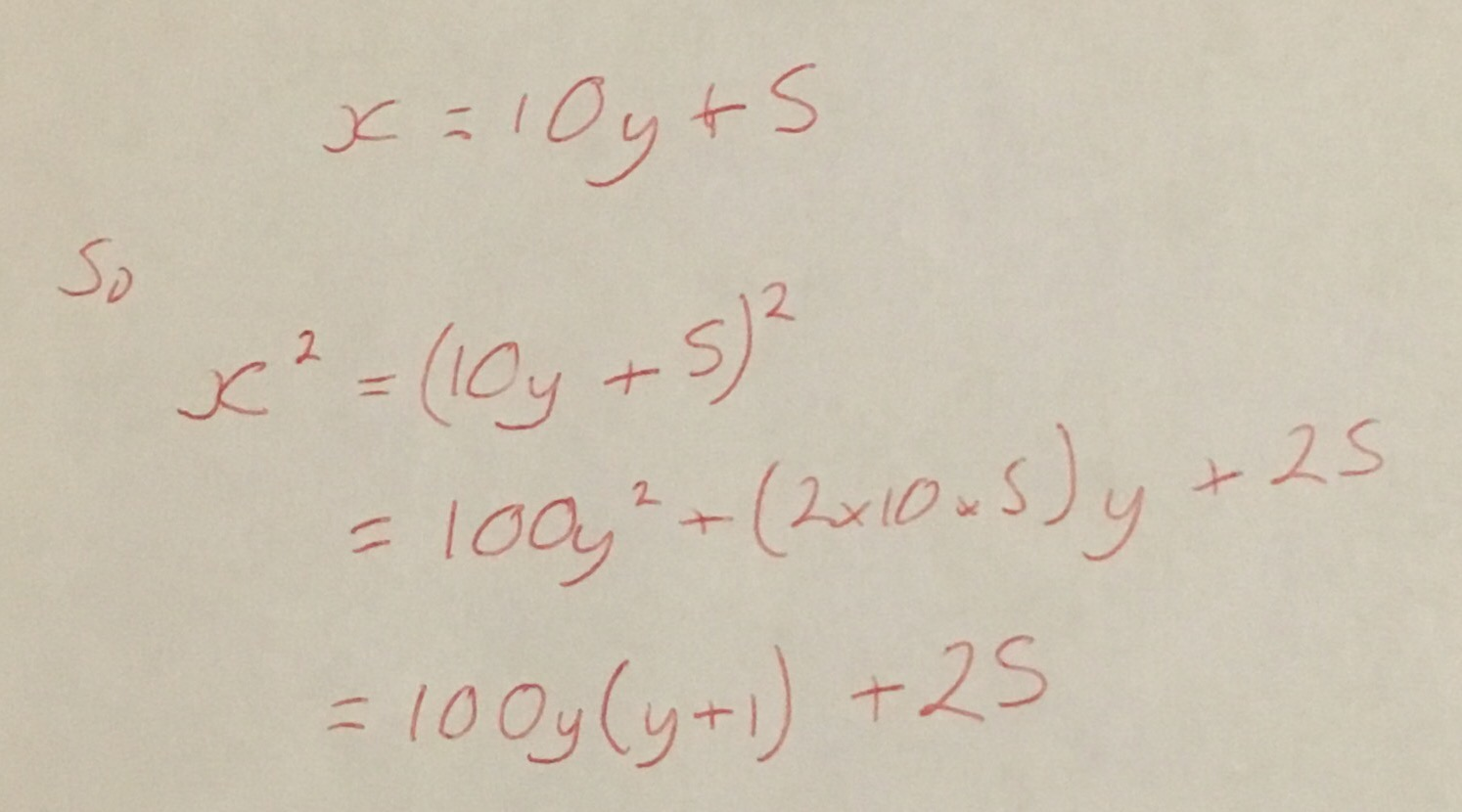Categories

# Some Tricks for UKMT Senior Team Challenges

This Wednesday was the Nottingham Regional Final of the UKMT Senior Team Maths Challenge and I was lucky enough to take my team of Sixth Formers who did really well (a very proud teacher here!).

I thought I would quickly share a couple of simple tricks for squaring numbers, which I am sure that most of you will know but I had never really had much of a need for things like this until I started preparing teams for this kind of thing.

The first one is for squaring numbers that end in 5. For any number ending in 5 take the digits before the five, call this $$n$$ and then multiply by $$n+1$$ before appending 25 to the result. For example for $$325^2$$ work out $$32 \times 33 = 1056$$ and so $$325^2 = 105625$$. To show why this works just consider the following.The last line of this is merely the product of $$y$$ and $$y+1$$ with 25 appended to the end.

Another trick makes use of algebraic identities to change the squaring of an arbitrary number into some easier squaring operations.

Consider $$a^2 = (a+b)(a-b) + b^2$$. This can be used to help us work out some squares, for example: